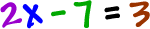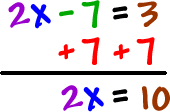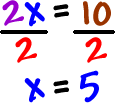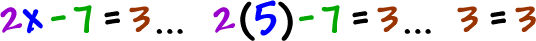Here's our ammunition:

 1) We can add or subtract a number from both sides of an equation. 2) We can multiply or divide both sides of an equation by a number. * And you always do them in this order.

Now, we'll need to do both in the same problem.

Check it out:

 SolveRemember our goal:  Get the x by himself!

There are two guys bugging the x...  -7 and 2.  The 2 is really locked on and the -7 is, kind of, hanging off...  So, we'll go after him first:Now, ditch the 2:Check it!Yep!